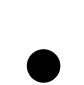Bag Of Cows

# Dog age in human years (Dog Years)

To calculate the age of a dog in human terms, we need to use two different multipliers, A and B.

For each of the dog's first two years of life, multiply its age by multiplier A
For all years after the first two, multiply by B

The value of A and B depends on the size of the dog (and in real life, the breed too).

Size of Dog Multiplier A Multiplier B
Small 12.5 5
Medium 10.5 6
Large 9.0 8

Here is what your program needs to do:

1. Input the size of dog - limit possible entries to "large", "medium" or "small"
2. Input the actual age of the dog in years
3. Set multiplier values for A and B based on size
4. Set a variable for age of dog up to two years
5. Set a variable for age of dog above two years
6. Calculate the human years for the first two years
7. Add on the human years for the remaining age above two years
8. Output the dog's real age and age in human years.

Test it with the following values:

Rover is a medium dog aged 5.5 years

A=10.5, B=6
2 * A = 21
6 * 3.5 = 21
Total = 42. Rover is 42 in dog years

Rex is a small dog aged 4 years
A = 12.5, B=5. Dog years = (12.5 * 2) + (5 * 2) = 35

Bella is a large dog aged 1.5 years.
A = 9, B=8. Dog years = 9 * 1.5 = 13.5